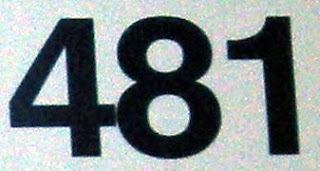## Wednesday, February 3, 2010

### 481

481 = 13 x 37.

481 has two representations as a sum of two squares: 481 = 92 + 202 = 152 + 162.

481 is the hypotenuse of two primitive Pythagorean triples: 4812 = 312 + 4802 = 3192 + 3602.

481 is an octagonal number.The Great Pyramid of Giza in Egypt is 481 feet high.

Source: WolframAlpha